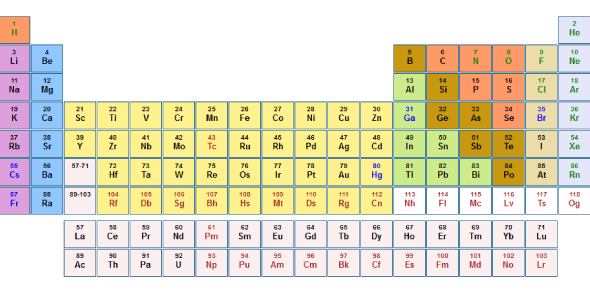# IT's All About Periodic Table Quiz

15 Questions | Total Attempts: 4922SettingsEven if you don’t know exactly what it means, we guarantee you’ve seen the periodic table before, whether it was on television or in your science class. But it’s time to finally take a closer look and figure out what it’s really all about in this one, in this “it’s all about periodic table quiz”!

• 1.
How many periods are on the periodic table?
• A.

18

• B.

25

• C.

7

• D.

30

• 2.
Which element is more reactive, Potassium or Krypton?
• A.

Potassium

• B.

Krypton

• C.

Both are the same

• D.

Neither, this is a trick question

• 3.
What is the atomic mass of the element in period 2, group 13?
• A.

5

• B.

10.81

• C.

13

• D.

26.982

• 4.
Which group contains the Noble gases?
• A.

15

• B.

16

• C.

17

• D.

18

• 5.
Which element has an atomic number of 79?
• A.

Gold

• B.

Bromine

• C.

Europium

• D.

Scienceium

• 6.
What side of the periodic table are the non-metals found on?
• A.

Left

• B.

Right

• C.

Top

• D.

Bottom

• 7.
What is the name of the element with the symbol "Pb?"
• A.

Peanut Butter

• B.

• C.

Platinum

• D.

What?

• 8.
Which of the following lists contain the elements that are most like Flourine?
• A.

Boron, Carbon, and Nitrogen

• B.

Lithium, Beryllium, and Boron

• C.

Chlorine, Bromine, and Iodine

• D.

Helium, Neon, and Argon

• 9.
Which element would be the better conductor of heat and electricity, Neon or Cobalt?
• A.

Cobalt

• B.

Neon

• C.

Both would be the same

• D.

Neither, this is a trick question

• 10.
What is the element symbol for the element in period 5, group 4?
• A.

Zirconium

• B.

Ruthorfordium

• C.

Zr

• D.

Rf

• 11.
What is the definition of ductile?
• A.

Something you can stretch into thin wires

• B.

Something you can mold and shape

• C.

Something you can put into the mail

• D.

Something you can find at the mall

• 12.
What do you use to find the number of protons in an element?
• A.

Atomic number

• B.

Atomic mass

• C.

Subtract the atomic mass from the atomic number

• D.

Subtract the atomic number from the atomic mass

• 13.
What do you use to find the number of neutrons in an element?
• A.

Atomic mass

• B.

Atomic number

• C.

Subtract the atomic mass from the atomic number

• D.

Subtract the atomic number from the atomic mass

• 14.
Which group on the periodic table has the largest number of elements?
• A.

Alkali metals

• B.

Alkaline metals

• C.

Transition metals

• D.

Halogens

• 15.
Image you discover a new element. After collecting your millions of dollars and your Nobel Prize, they ask you to decide where you would like to put it on the Periodic Table. Using your knowledge of how it is organized, describe where you would put your element and explain why. Please give your element a name and describe its charactoristics to help you explain where you will put it on the table.
Related TopicsBack to top